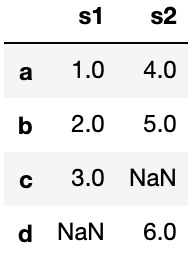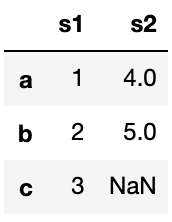# [Python Cookbook]Pandas: How to increase columns for DataFrame?Join/Concat

## 1. Combine Two Series

series1=pd.Series([1,2,3],name='s1')
series2=pd.Series([4,5,6],name='s2')
df = pd.concat([series1, series2], axis=1)

Out:series1=pd.Series([1,2,3],index=['a','b','c'],name='s1')
series2=pd.Series([4,5,6],index=['a','b','d'],name='s2')
df = pd.concat([series1, series2], axis=1)

Out:Note: Two series must have names.

## 2. Add a series to a data frame

df=pd.DataFrame([1,2,3],index=['a','b','c'],columns=['s1'])
s2=pd.Series([4,5,6],index=['a','b','d'],name='s2')
df['s2']=s2

Out:This method is equivalant to left join:

d2.join(s2,how='left',inplace=True)

To get the same result as Part 1, we can use outer join:

d2.join(s2,how='outer',inplace=True)

posted @ 2020-02-28 04:51  Sherrrry  阅读(156)  评论(0编辑  收藏  举报